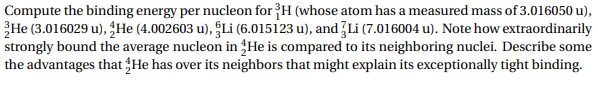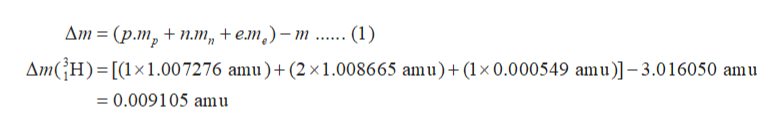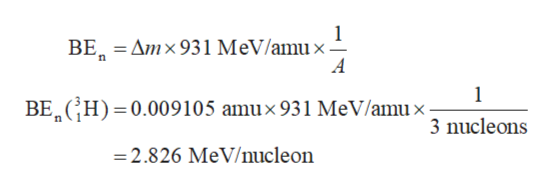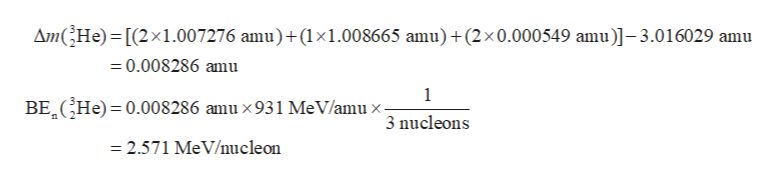# Compute the binding energy per nucleon for H (whose atom has a measured mass of 3.016050 u)3He (3.016029 u),JHe (4.002603 u), Li (6.015123 u), and 3Li (7.016004 u). Note how extraordinarilystrongly bound the average nucleon in 4He is compared to its neighboring nuclei. Describe somethe advantages that4He has over its neighbors that might explain its exceptionally tight binding

Question
11 viewshelp_outlineImage TranscriptioncloseCompute the binding energy per nucleon for H (whose atom has a measured mass of 3.016050 u) 3He (3.016029 u),JHe (4.002603 u), Li (6.015123 u), and 3Li (7.016004 u). Note how extraordinarily strongly bound the average nucleon in 4He is compared to its neighboring nuclei. Describe some the advantages that4He has over its neighbors that might explain its exceptionally tight binding fullscreen
check_circle

Step 1

The mass defect (Δm) is calculated using equation (1) in which m is the given mass of atom, p is the number protons, n is the number of neutrons, e is the number of electrons, mp is the mass of proton, mn is the mass of neutron and me is the mass of electron. The mass defect for 31H is calculated as follows:help_outlineImage TranscriptioncloseДт %3D (рт, + пт, + ет,) - т Дт(;H)3D[(1x1.007276 amu) + (2х1.008665 amu)+ (1x0.000549 аmu)]-3.016050 amu (1) 3D 0.009105 аmu fullscreen
Step 2

The binding energy per nucleon (BEn) for 31H is calculated using equation (2) in which A is the number of nucleons.help_outlineImage Transcriptionclose1 BE Amx931 MeV/amux- 1 BE CH) 0.009105 amux 931 MeV/amux- 3 nucleons =2.826 MeV/mucleon fullscreen
Step 3

Similarly, the mass defect and the binding energy for 3...help_outlineImage TranscriptioncloseAmGHe) [(2x1.007276 amu)+(1x1.008665 amu)+(2x0.000549 amu)]-3.016029 amu 0.008286 amu 1 He) 0.008286 amu x 931 MeV/amu x BE 3 nucleons = 2.571 MeV/nucle on fullscreen

### Want to see the full answer?

See Solution

#### Want to see this answer and more?

Solutions are written by subject experts who are available 24/7. Questions are typically answered within 1 hour.*

See Solution
*Response times may vary by subject and question.
Tagged in

### Chemistry